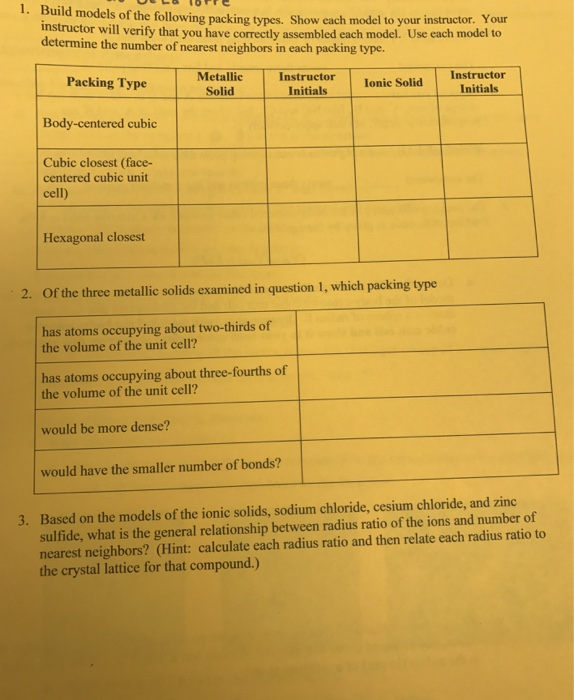# Question & Answer: Build models of the following packing types. Show each model to your instructor. Your…..Build models of the following packing types. Show each model to your instructor. Your instructor will verify that you have correctly assembled each model. Use each model to determine the number of nearest neighbors in each packing type. Of the three metallic solids examined in question 1, which packing type has atoms occupying about two-thirds of the volume of the unit cell? has atoms occupying about three-fourths of the volume of the unit cell? would be more dense? would have the smaller number of bonds? Based on the models of the ionic solids, sodium chloride, cesium chloride, and zinc sulfide, what is the general relationship between radius ratio of the ions and number of nearest neighbors?

bcc2. —> in bcc, atoms occupy two-third volume of the unit cell i.e. packing efficiency = 68%

—> in fcc or hcp, atoms occupy three-fourthvolume of the unit cell i.e. packing efficiency = 74%

—> fcc or hcp would be denser than bcc

Don't use plagiarized sources. Get Your Custom Essay on
Question & Answer: Build models of the following packing types. Show each model to your instructor. Your…..
GET AN ESSAY WRITTEN FOR YOU FROM AS LOW AS \$13/PAGE

—-> bcc have lesser no. of bonds

3.—-> r+/r <0.155 linear CN = 2

—-> r+/r =0.155-0.225 planar CN = 3

—–> r+/r = 0.225 – 0.414 tetrahedral CN = 4

—–> r+/r = 0.414 – 0.732 octahedral CN = 6

—–> r+/r = 0.732 – 1.0 cubic CN = 8